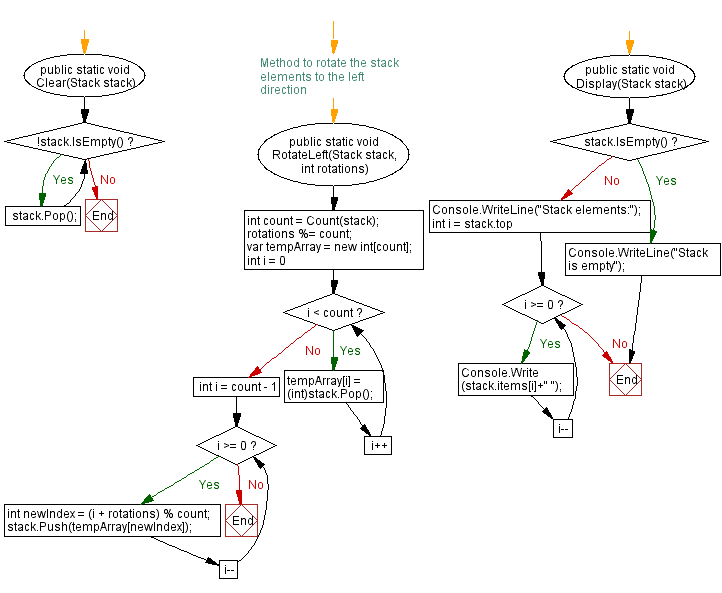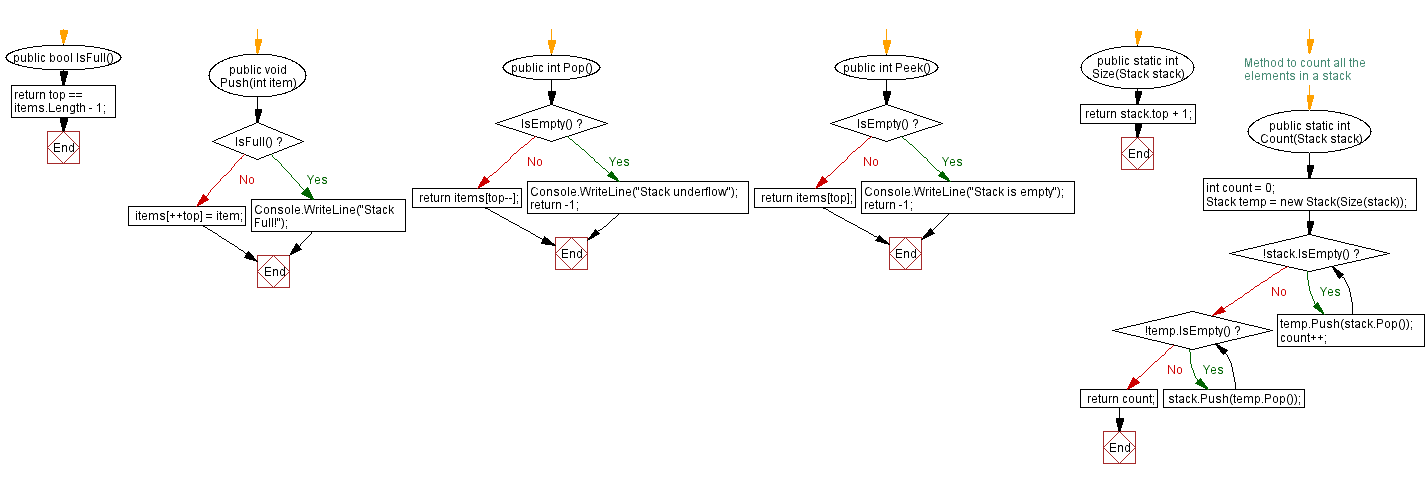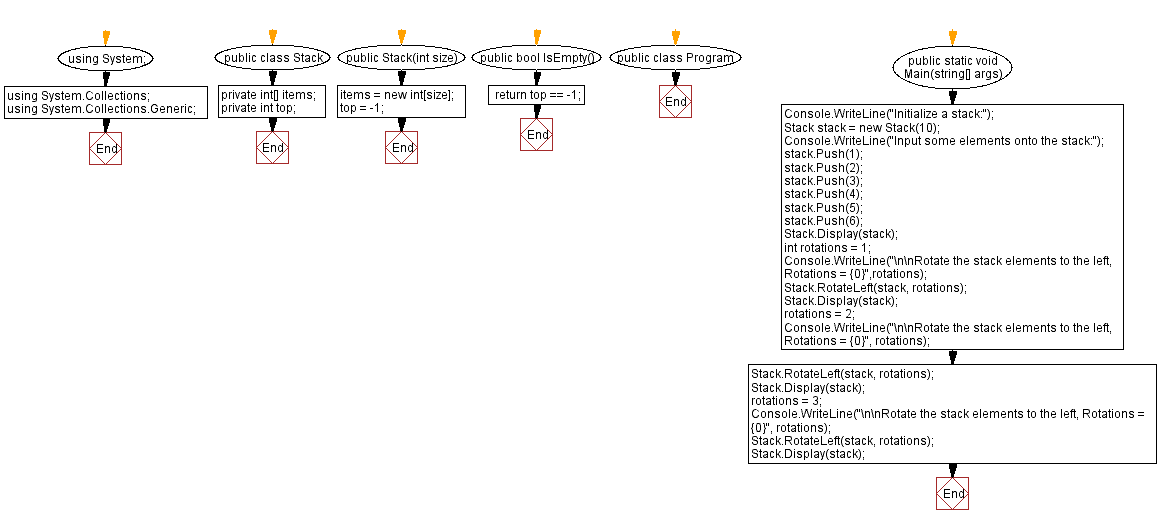﻿ C# - Rotate the stack elements to the left

# C# Sharp Stack Exercises: Rotate the stack elements to the left

## C# Sharp Stack: Exercise-14 with Solution

Write a C# program to rotate the stack elements to the left direction.

Sample Solution:

C# Code:

``````using System;
using System.Collections;
using System.Collections.Generic;
public class Stack
{
private int[] items;
private int top;

public Stack(int size)
{
items = new int[size];
top = -1;
}

public bool IsEmpty()
{
}

public bool IsFull()
{
}

public void Push(int item)
{
if (IsFull())
{
Console.WriteLine("Stack Full!");
return;
}

items[++top] = item;
}

public int Pop()
{
if (IsEmpty())
{
Console.WriteLine("Stack underflow");
return -1;
}

return items[top--];
}

public int Peek()
{
if (IsEmpty())
{
Console.WriteLine("Stack is empty");
return -1;
}

return items[top];
}

public static int Size(Stack stack)
{
return stack.top + 1;
}

// Method to count all the elements in a stack
public static int Count(Stack stack)
{
int count = 0;

Stack temp = new Stack(Size(stack));

while (!stack.IsEmpty())
{
temp.Push(stack.Pop());
count++;
}

while (!temp.IsEmpty())
{
stack.Push(temp.Pop());
}

return count;
}

public static void Clear(Stack stack)
{
while (!stack.IsEmpty())
{
stack.Pop();
}
}

// Method to rotate the stack elements to the left direction

public static void RotateLeft(Stack stack, int rotations)
{
int count = Count(stack);
rotations %= count;

var tempArray = new int[count];

for (int i = 0; i < count; i++)
{
tempArray[i] = (int)stack.Pop();
}

for (int i = count - 1; i >= 0; i--)
{
int newIndex = (i + rotations) % count;
stack.Push(tempArray[newIndex]);
}
}
public static void Display(Stack stack)
{
if (stack.IsEmpty())
{
Console.WriteLine("Stack is empty");
return;
}
Console.WriteLine("Stack elements:");
for (int i = stack.top; i >= 0; i--)
{
Console.Write(stack.items[i]+" ");
}
}

}

public class Program
{
public static void Main(string[] args)
{
Console.WriteLine("Initialize a stack:");
Stack stack = new Stack(10);
Console.WriteLine("Input some elements onto the stack:");
stack.Push(1);
stack.Push(2);
stack.Push(3);
stack.Push(4);
stack.Push(5);
stack.Push(6);
Stack.Display(stack);
int rotations = 1;
Console.WriteLine("\n\nRotate the stack elements to the left, Rotations = {0}",rotations);
Stack.RotateLeft(stack, rotations);
Stack.Display(stack);
rotations = 2;
Console.WriteLine("\n\nRotate the stack elements to the left, Rotations = {0}", rotations);
Stack.RotateLeft(stack, rotations);
Stack.Display(stack);
rotations = 3;
Console.WriteLine("\n\nRotate the stack elements to the left, Rotations = {0}", rotations);
Stack.RotateLeft(stack, rotations);
Stack.Display(stack);
}
}
``````

Sample Output:

```Initialize a stack:
Input some elements onto the stack:
Stack elements:
6 5 4 3 2 1

Rotate the stack elements to the left, Rotations = 1
Stack elements:
5 4 3 2 1 6

Rotate the stack elements to the left, Rotations = 2
Stack elements:
3 2 1 6 5 4

Rotate the stack elements to the left, Rotations = 3
Stack elements:
6 5 4 3 2 1
```

Flowchart:C# Sharp Code Editor:

Improve this sample solution and post your code through Disqus

What is the difficulty level of this exercise?

Test your Programming skills with w3resource's quiz.

﻿